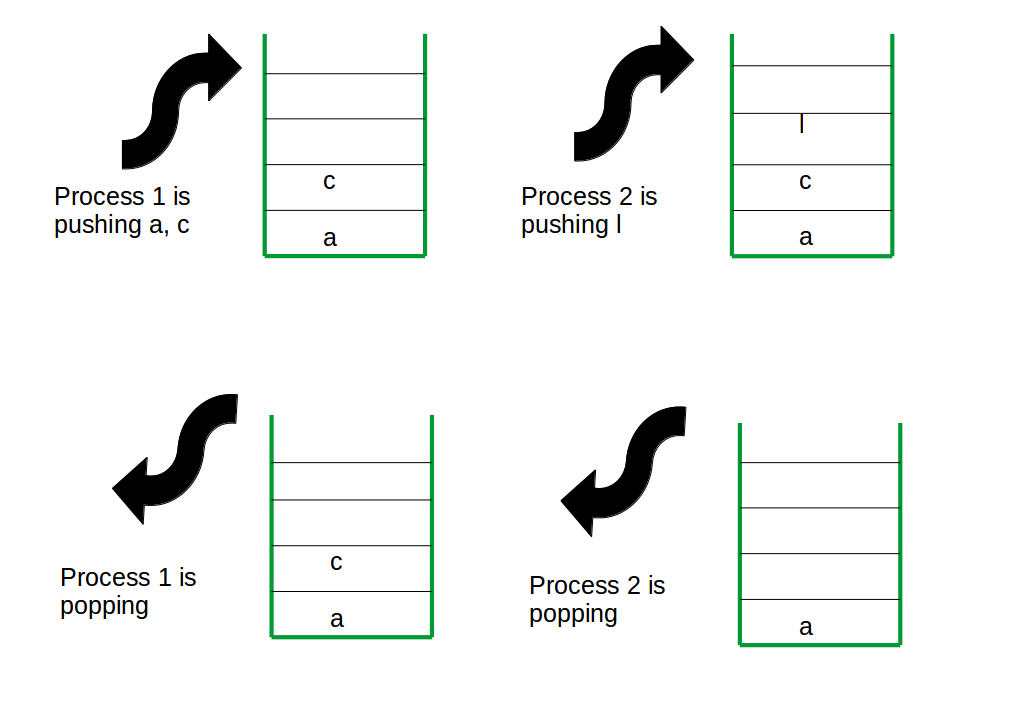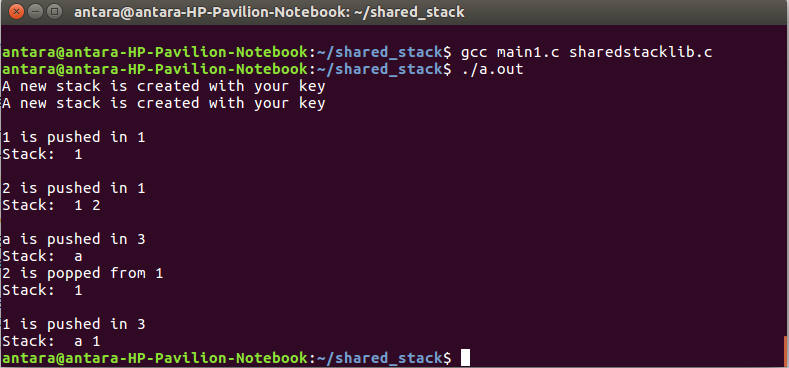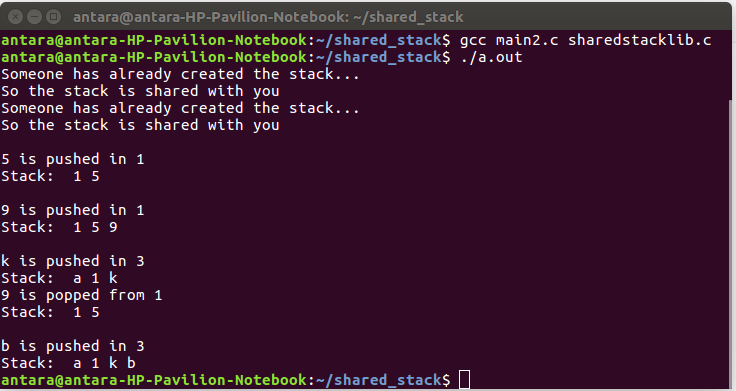# Inter-process Communication using a shared stack

Inter Process Communication through shared memory is a concept where two or more process can access the common memory.the communication is done via this shared memory where changes made by one process can be viewed by anther process. So, we can use one stack as a shared memory, where users(processes) sharing the stack (stacks) can push or pop elements. They can create one new shared stack and can also delete them.Now, it is time for header file. If there is one header (.h extension) and one library file then all the processes can use those library functions so they don’t need to write the code for each function. So, there is 3 programs.
2. sharedstacklib.c– Library file for stacklib.h.
3. main.c – The users code.

We can use 4 functions for the shared stack operation.
1.shstackpush()– For push the elements.
2.shstackpop()– For pop out the last element.
3.shstackget()– User will enter one key and a shared stack will be created with that key. All the push, pop or remove function will be done by that particular key for particular stack. If any user enter the same key it will share the stack with the user/ users who previously created the stack with that key.
4.shstackrm()– For removing the stack.

Some IPC system calls has been used-
shmget()– Creates shared memory segment.
shmat(), shmdt()– Attaches and detaches with the shared memory.
shmctl()– Removes the shared memory segment.
semget()– Creates semaphore.
semctl()– Controls semaphore operations.

1. stacklib.h – Header file. Code is given below

 `// C Program for header file of a shared stack ` `#include ` `#include ` `#include ` `#include ` `#include ` `#include ` `#include ` `#include ` `#include ` `#include ` ` `  `#ifndef _MYLIB_H_ ` `#define _MYLIB_H_ ` ` `  `// structure for stack descriptor ` `struct` `stack_desc { ` `    ``key_t stkey; ``// key for the stack ` `    ``int` `data_size; ``// integer or character ` `    ``int` `stack_size; ` `    ``int` `top; ` `    ``int` `ele_no; ` `    ``bool` `free``; ``// stack is used by any process ot not ` `}; ` `typedef` `struct` `stack_desc stack_desc; ` ` `  `extern` `void` `shstackget(key_t mykey, ``int` `data_size, ``int` `stack_size); ` `extern` `void` `shstackpush(key_t key, ``int` `ele); ` `extern` `void` `shstackpop(key_t key); ` `extern` `void` `shstackrm(key_t key); ` ` `  `#endif `

2. sharedstacklib.c – Code is given below

 `// C Program for sharedstacklib.c ` `#include "stacklib.h" ` `#define NO_SEM 1 ` ` `  `// P(s) for adding 1 with the current semaphore value ` `#define P(s) semop(s, &Pop, 1); ` ` `  `// v(s) for reducing 1 with the current semaphore value ` `#define V(s) semop(s, &Vop, 1); ` ` `  `struct` `sembuf Pop; ` `struct` `sembuf Vop; ` ` `  `int``* k; ` `struct` `stack_desc (*shared_stacks); ` `void` `shstackget(key_t mykey, ``int` `data_size, ``int` `stack_size) ` `{ ` `    ``int` `status; ` ` `  `    ``union` `semun { ` `        ``int` `val; ``/* Value for SETVAL */` `        ``struct` `semid_ds* buf; ``/* Buffer for IPC_STAT, IPC_SET */` `        ``unsigned ``short``* array; ``/* Array for GETALL, SETALL */` `        ``struct` `seminfo* __buf; ``/* Buffer for IPC_INFO (Linux-specific) */` `    ``} setvalArg; ` ` `  `    ``Pop.sem_num = 0; ` `    ``Pop.sem_op = -1; ` `    ``Pop.sem_flg = SEM_UNDO; ` ` `  `    ``Vop.sem_num = 0; ` `    ``Vop.sem_op = 1; ` `    ``Vop.sem_flg = SEM_UNDO; ` ` `  `    ``// creating the key for the sharedstack descriptor ` `    ``key_t key = ftok(``"/home/antara/shared_stack/sharedstacklib.c"``, 1); ` `    ``if` `(key == -1) { ` `        ``perror``(``"ftok() failed"``); ` `        ``exit``(1); ` `    ``} ` ` `  `    ``// shmid for the stack descriptor ` `    ``int` `shmid = shmget(key, ``sizeof``(``struct` `stack_desc), IPC_CREAT | 0777); ` ` `  `    ``shared_stacks = shmat(shmid, NULL, 0); ` `    ``int` `i; ` `    ``key_t newkey, keys; ` `    ``key_t y = ftok(``"/home/antara/stackkey.c"``, 1); ` ` `  `    ``// for attachment of a shared array of keys ` `    ``int` `shmidt = shmget(y, ``sizeof``(``int``), IPC_CREAT | 0777); ` ` `  `    ``k = shmat(shmidt, NULL, 0); ` `    ``for` `(i = 0; i < 10; i++) { ` `        ``if` `(k[i] == mykey && shared_stacks[i]->``free` `== ``true``) ``// for already used key ` `        ``{ ` `            ``printf``(``"Someone has already created the stack...\nSo the stack is shared with you\n"``); ` `            ``int` `semid = semget(shared_stacks[i]->stkey, NO_SEM, IPC_CREAT | 0777); ` `            ``break``; ` `        ``} ` `        ``else` `if` `(k[i] != mykey && shared_stacks[i]->``free` `== ``false``) ``// for new key ` `        ``{ ` `            ``shared_stacks[i]->``free` `= ``true``; ` ` `  `            ``k[i] = mykey; ` `            ``newkey = i + 1; ` `            ``char` `file[] = ``"f_.txt"``; ` `            ``file = ``'0'` `+ (newkey - 1); ` `            ``FILE``* fp; ` `            ``fp = ``fopen``(file, ``"w"``); ``// creating one .txt file for ftok() of different stack segments ` `            ``fclose``(fp); ` `            ``char` `keyp; ` `            ``strcpy``(keyp, ``"/home/antara/shared_stack/"``); ` ` `  `            ``strcat``(keyp, file); ` ` `  `            ``keys = ftok(keyp, 1); ` `            ``if` `(keys == -1) { ` `                ``perror``(``"ftok() failed"``); ` `                ``exit``(1); ` `            ``} ` ` `  `            ``/*updating the stack_desc*/` `            ``shared_stacks[i]->stkey = keys; ` `            ``shared_stacks[i]->top = -1; ` `            ``shared_stacks[i]->data_size = data_size; ` `            ``shared_stacks[i]->stack_size = stack_size; ` `            ``shared_stacks[i]->ele_no = 0; ` `            ``printf``(``"A new stack is created with your key \n"``); ` `            ``setvalArg.val = 1; ` `            ``int` `semid = semget(keys, NO_SEM, IPC_CREAT | 0777); ` `            ``status = semctl(semid, 0, SETVAL, setvalArg); ``// making semaphore value 1 ` `            ``if` `(status == -1) { ` `                ``perror``(``"semctl() failed"``); ` `                ``exit``(1); ` `            ``} ` `            ``break``; ` `        ``} ` `    ``} ` `} ` ` `  `// For pushing elements in the stack ` `void` `shstackpush(key_t key, ``int` `ele) ` `{ ` `    ``int` `i, j = -1; ` `    ``for` `(i = 0; i < 10; i++) { ` `        ``if` `(k[i] == key) ``// valid key ` `        ``{ ` `            ``j = i; ` `            ``break``; ` `        ``} ` `    ``} ` `    ``int` `semid; ` ` `  `    ``if` `(j == -1) ``// invalid key ` `    ``{ ` `        ``printf``(``"No stack with your key\nTry again with different key\n"``); ` `    ``} ` `    ``else` `if` `(j != -1) { ` `        ``P(semid); ` ` `  `        ``// for integer ` `        ``if` `(shared_stacks[j]->data_size == 4) { ` `            ``int``* stack; ` `            ``key_t keys = shared_stacks[j]->stkey; ` `            ``int` `shmid = shmget(keys, ``sizeof``(``int``) * shared_stacks[j]->stack_size, IPC_CREAT | 0777); ` `            ``int` `h = ele; ` `            ``stack = shmat(shmid, NULL, 0); ` `            ``int` `top = shared_stacks[j]->top; ` `            ``if` `(top == shared_stacks[j]->stack_size - 1) ``// empty stack ` `            ``{ ` `                ``printf``(``"No elements can be pushed\n"``); ` `            ``} ` `            ``else` `if` `(top < shared_stacks[j]->stack_size) { ` `                ``top++; ` `                ``stack[top] = h; ` `                ``printf``(``"\n%d is pushed in %d\n"``, stack[top], key); ` ` `  `                ``shared_stacks[j]->top = top; ` `                ``shared_stacks[j]->ele_no = top + 1; ` `            ``} ` `            ``printf``(``"Stack:  "``); ` `            ``for` `(i = 0; i <= top; i++) { ` `                ``printf``(``"%d "``, stack[i]); ` `            ``} ` `            ``printf``(``"\n"``); ` `            ``shmdt(stack); ` `        ``} ` ` `  `        ``// for character ` `        ``else` `if` `(shared_stacks[j]->data_size == 1) { ` `            ``char``* stack; ` `            ``key_t keys = shared_stacks[j]->stkey; ` `            ``int` `shmid = shmget(keys, ``sizeof``(``char``) * shared_stacks[j]->stack_size, IPC_CREAT | 0777); ` `            ``int` `semid = semget(keys, NO_SEM, IPC_CREAT | 0777); ` ` `  `            ``stack = shmat(shmid, NULL, 0); ` `            ``int` `top = shared_stacks[j]->top; ` `            ``if` `(top == shared_stacks[j]->stack_size - 1) ``// empty stack ` `            ``{ ` `                ``printf``(``"No elements can be pushed\n"``); ` `            ``} ` ` `  `            ``else` `if` `(top < shared_stacks[j]->stack_size) { ` `                ``top++; ` `                ``stack[top] = ele; ` `                ``printf``(``"\n%c is pushed in %d\n"``, stack[top], key); ` ` `  `                ``shared_stacks[j]->top = top; ` `                ``shared_stacks[j]->ele_no = top + 1; ` `            ``} ` ` `  `            ``printf``(``"Stack:  "``); ` `            ``for` `(i = 0; i <= top; i++) { ` `                ``printf``(``"%c "``, stack[i]); ` `            ``} ` `            ``printf``(``"\n"``); ` `            ``shmdt(stack); ` `        ``} ` `        ``V(semid); ` `    ``} ` `} ` ` `  `// Function for popping the last element out ` `void` `shstackpop(key_t key) ` `{ ` `    ``int` `i, j = -1; ` `    ``for` `(i = 0; i < 10; i++) { ` `        ``if` `(k[i] == key) ``// valid key ` `        ``{ ` `            ``j = i; ` `            ``break``; ` `        ``} ` `    ``} ` `    ``int` `semid; ` `    ``if` `(j == -1) ``// invalid key ` `    ``{ ` `        ``printf``(``"No stack with your key\nTry again with different key\n"``); ` `    ``} ` `    ``else` `if` `(j != -1) { ` `        ``P(semid); ` `        ``if` `(shared_stacks[j]->data_size == 4) { ` `            ``key_t keys = shared_stacks[j]->stkey; ` `            ``int``* stack; ` `            ``int` `shmid = shmget(keys, ``sizeof``(``int``) * shared_stacks[j]->stack_size, IPC_CREAT | 0777); ` `            ``semid = semget(keys, NO_SEM, IPC_CREAT | 0777); ` ` `  `            ``stack = shmat(shmid, NULL, 0); ` `            ``int` `top = shared_stacks[j]->top; ` `            ``if` `(top == -1) { ` `                ``printf``(``"No element can be popped \n"``); ` `            ``} ` `            ``else` `if` `(top >= -1) { ` `                ``int` `a = stack[top]; ` `                ``top--; ` `                ``printf``(``"%d is popped from %d\n"``, a, key); ` `                ``shared_stacks[j]->top = top; ` `                ``shared_stacks[j]->ele_no = top + 1; ` `            ``} ` `            ``printf``(``"Stack:  "``); ` `            ``for` `(i = 0; i <= top; i++) { ` `                ``printf``(``"%d "``, stack[i]); ` `            ``} ` `            ``printf``(``"\n"``); ` `            ``shmdt(stack); ` `        ``} ` `        ``else` `if` `(shared_stacks[j]->data_size == 1) { ` `            ``key_t keys = shared_stacks[j]->stkey; ` `            ``char``* stack; ` `            ``int` `shmid = shmget(keys, ``sizeof``(``char``) * shared_stacks[j]->stack_size, IPC_CREAT | 0777); ` `            ``semid = semget(keys, NO_SEM, IPC_CREAT | 0777); ` `            ``stack = shmat(shmid, NULL, 0); ` `            ``int` `top = shared_stacks[j]->top; ` `            ``if` `(top == -1) { ` `                ``printf``(``"No element can be popped \n"``); ` `            ``} ` `            ``else` `if` `(top >= -1) { ` `                ``int` `a = stack[top]; ` `                ``top--; ` `                ``printf``(``"%c is popped from %d\n"``, a, key); ` `                ``shared_stacks[j]->top = top; ` `                ``shared_stacks[j]->ele_no = top + 1; ` `            ``} ` `            ``printf``(``"Stack:  "``); ` `            ``for` `(i = 0; i <= top; i++) { ` `                ``printf``(``"%c "``, stack[i]); ` `            ``} ` `            ``printf``(``"\n"``); ` `            ``shmdt(stack); ` `        ``} ` `        ``V(semid); ` `    ``} ` `} ` ` `  `// Function for removing the stack ` `void` `shstackrm(key_t key) ` `{ ` `    ``int` `i; ` `    ``int` `j = -1; ` `    ``for` `(i = 0; i < 10; i++) { ` `        ``if` `(k[i] == key) ``// valid key ` `        ``{ ` `            ``j = i; ` `            ``break``; ` `        ``} ` `    ``} ` `    ``if` `(j == -1) ``// invalid key ` `    ``{ ` `        ``printf``(``"No stack with your key\nTry again with different key\n"``); ` `    ``} ` ` `  `    ``else` `if` `(j != -1) { ` `        ``key_t keys = shared_stacks[j]->stkey; ` `        ``int` `shmid; ` `        ``if` `(shared_stacks[j]->data_size == 4) { ` `            ``shmid = shmget(keys, ``sizeof``(``int``) * shared_stacks[j]->stack_size, IPC_CREAT | 0777); ` `        ``} ` `        ``else` `if` `(shared_stacks[j]->data_size == 1) { ` `            ``shmid = shmget(keys, ``sizeof``(``char``) * shared_stacks[j]->stack_size, IPC_CREAT | 0777); ` `        ``} ` `        ``shared_stacks[j]->``free` `= ``false``; ` `        ``shared_stacks[j]->top = -1; ` `        ``k[j] = 0; ` `        ``shmctl(shmid, IPC_RMID, NULL); ``// removing the stack ` `        ``printf``(``"Stack is removed\n"``); ` `    ``} ` `} `

3. main1.c -Process no 1 call1 the functions.
Code is given below

 `// C Program for process1 ` `#include "stacklib.h" ` `#include ` ` `  `int` `main() ` `{ ` ` `  `    ``shstackget(1, 4, 10); ` `    ``shstackget(3, 1, 6); ` `    ``shstackpush(1, 1); ` `    ``shstackpush(1, 2); ` `    ``shstackpush(3, ``'a'``); ` `    ``shstackpop(1); ` `    ``shstackpush(3, ``'1'``); ` ` `  `    ``return` `0; ` `} `

3. main2.c -Process no 2 calls the functions.
Code is given below

 `// C Program for process2 ` `#include "stacklib.h" ` `#include ` ` `  `int` `main() ` `{ ` `    ``shstackget(1, 4, 10); ` `    ``shstackget(3, 1, 6); ` `    ``shstackpush(1, 5); ` `    ``shstackpush(1, 9); ` `    ``shstackpush(3, ``'k'``); ` `    ``shstackpop(1); ` `    ``shstackpush(3, ``'b'``); ` ` `  `    ``return` `0; ` `} `

You can compile the code using

`gcc main1.c sharedstacklib.c`
`gcc main2.c sharedstacklib.c`

Output:
After executing main1.cAfter executing main2.cGeeksforGeeks has prepared a complete interview preparation course with premium videos, theory, practice problems, TA support and many more features. Please refer Placement 100 for details

My Personal Notes arrow_drop_upCheck out this Author's contributed articles.

If you like GeeksforGeeks and would like to contribute, you can also write an article using contribute.geeksforgeeks.org or mail your article to contribute@geeksforgeeks.org. See your article appearing on the GeeksforGeeks main page and help other Geeks.

Please Improve this article if you find anything incorrect by clicking on the "Improve Article" button below.

Improved By : Akanksha_Rai

Article Tags :
Practice Tags :

1

Please write to us at contribute@geeksforgeeks.org to report any issue with the above content.